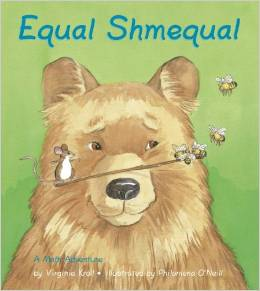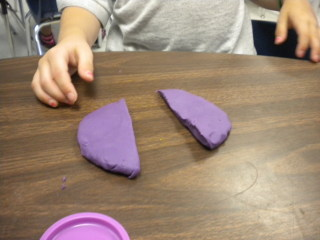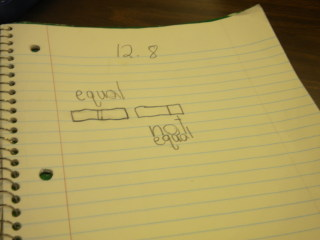# Equal Parts

11 teachers like this lesson
Print Lesson

## Objective

SWBAT identify equal and unequal parts (or shares) in two-dimensional shapes.

#### Big Idea

Equal Schmequal! In this lesson students will examine the parts of a shape and determine if they are equal or unequal.

## Activating Strategy

10 minutes

I begin this lesson by reading aloud, “Equal Schmequal”.  This story is highly engaging for students and begins to build the vocabulary for equal and unequal.After reading the story, I draw a circle on the board and ask a student to draw a line to show two parts. I then draw a trapezoid, and invite a different student to draw a line to show two triangles. Finally, draw a rectangle, and have another student draw a line to show two squares. Reinforce that the lines drawn show parts of the shapes.

A real-life situation for this concept is sharing food. Circular shapes (pizzas or cookies), rectangular shapes (candy bars or graham crackers), or pieces that are part of a group (M&Ms or cheerios) could be used to show students how to divide into equal parts so that everyone can have the same size piece.  Deciding whether a shape (or a whole) has been partitioned into equal or unequal parts is the beginning of children’s experience with fractions (1.G.A.3). You'll see in the next section that this approach helps children begin to develop a conceptual basis for understanding fractions.

## Teaching Strategies

15 minutes

To help the students visualize equal shares, I hand out play-doh to each student.  I instruct them to make a circle with their play-doh.  I then tell them to pretend their circle is a cookie and ask them how they would share it with their friend.

• How would you share this circle with your friend, if it was a cookie? Remember that we want to share it so that each person has an equal size piece of the cookie.  (I would cut it.  I would break it.)

I then have students cut or break their play-doh cookie.  I then have them place the pieces on top of each other and have them discuss with their shoulder partner whether they are equal or unequal shares.After discussing equal shares with play-doh, I hand each student 10 connecting cubes.  I instruct them to put their connecting cubes into a cube train that has the same number of cubes.  This is another concrete example that will allow students to visualize that equal shares means that they are the same size or same number.

I then direct students to look at the first slide of the Equal Shares.ppt and read the following directions aloud:

Draw a line to show two triangles in the square.

• How many sides and vertices does a triangle have? (3 sides and 3 vertices

I want to help children visualize how to show two triangles in the square:

• Where will you draw your line? (I will draw my line from one top vertex to the bottom vertex on the opposite side.)

I then direct children’s attention to the second square and read the following directions aloud:

Draw lines to show three triangles in the square.

As I lead children through this process, I am guiding them to understand that the three triangles will not all be the same size.

• Start with a line like the one you drew in the first square. How can you draw another line to show three triangles? (I can split one triangle in two by drawing a line from a bottom vertex to the middle of the first line.)
• How can you draw three triangles in a different way?

Finally, I allow volunteers to show any different ways they made triangles.

## Independent Practice

30 minutes

I work through the example on the top of the Equal Parts_worksheet.docx with children to connect the vocabulary terms to the shapes shown. I read the question in the cloud and invite children to share their answers.

• How many equal parts does the top square have? (2 equal parts)
• Can a shape have more than two equal parts? Explain. (Yes. The bottom square shows four equal parts.)
• How are equal parts different from unequal parts? (Equal parts are the same size. Unequal parts would be different sizes.)

I then release students to complete the worksheet on their own.

## Closing/Summarizing

5 minutes

To close out the lesson, I have students draw 2 rectangles in their math journal. I then instruct them to divide one rectangle into equal shares and the other into unequal shares.